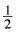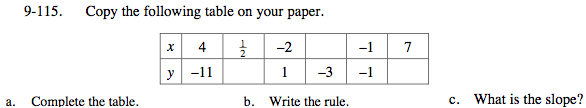### Home > MC2 > Chapter 9 > Lesson 9.3.3 > Problem9-115

9-115.
1. Copy the following table on your paper. 9-115 HW eTool (Desmos). Homework Help ✎

 x 4–2 –1 7 y –11 1 –3 –1
1. Complete the table.

2. Write the rule.

3. What is the slope?Use pattern between different coordinates to help you fill in the table.

See problem 9-82 for help in finding the rule of the table.

Rule: y = −2x − 3
What would the slope be based on this equation?

It might be easier to use the points (−2, 1) and (−1, −1) to find the slope.

Explore the problem with the eTool below.
Click the link at right for the full version of the eTool: MC2 9-115 HW eTool